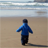# QlikView App Dev

Discussion Board for collaboration related to QlikView App Development.

cancel
Showing results for
Did you mean:Creator

## Creating a Heat Map

Hi Experts,

I am trying to create an Heat map with below requirement.

If 1<Sum(Measure)<=0.75 then the cell should be green

0.75<Sum(Measure)<=0.5 then the cell should be Yellow

0.5<Sum(Measure)<=0.1 then the cell should be Red

Other values should left out with Grey.

When I have tried to create I could see different colors are being applied in the cell background.

I have attached the data and Sample app. Could you please check and help me in getting this done.

Thanks

S k

2 RepliesMVP

Have you tried like:

=If( 1<Sum(Measure) and Sum(Measure)>=0.75, green(),

if(Sum(Measure)>=0.5, yellow(),

if(Sum(Measure)>=0.1, lightred())))MVP

How is your expected result different from what you already have achieved?